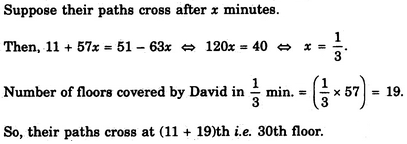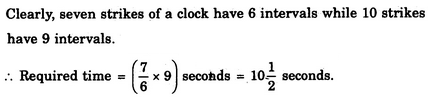# Verbal Reasoning - Arithmetic Reasoning

Exercise : Arithmetic Reasoning - Section 2
1.
A student got twice as many sums wrong as he got right. If he attempted 48 sums in all, how many did he solve correctly ?
12
16
18
24
Explanation:

Suppose the boy got x sums right and 2x sums wrong.

Then, x + 2x = 483x = 48x = 16.

2.
David gets on the elevator at the 11th floor of a building and rides up at the rate of 57 floors per minute. At the same time, Albert gets on an elevator at the 51st floor of the same building and rides down at the rate of 63 floors per minute. If they continue travelling at these rates, then at which floor will their paths cross ?
19
28
30
37
Explanation:3.
I have a few sweets to be distributed. If I keep 2, 3 or 4 in a pack, I am left with one sweet. If I keep 5 in a pack, I am left with none. What is the minimum number of sweets I have to pack and distribute ?
25
37
54
65
Explanation:

Clearly, the required number would be such that it leaves a remainder of 1 when divided by 2, 3 or 4 and no remainder when divided by 5. Such a number is 25.

4.
If a clock takes seven seconds to strike seven, how long will it take to strike ten ?
7 seconds
9 seconds
10 seconds
None of these
Explanation:5.
In a group of cows and hens, the number of legs are 14 more than twice the number of heads. The number of cows is
5
7
10
12
Then, 4x + 2y = 2 (x + y) + 144x + 2y = 2x + 2y + 142x = 14x = 7.